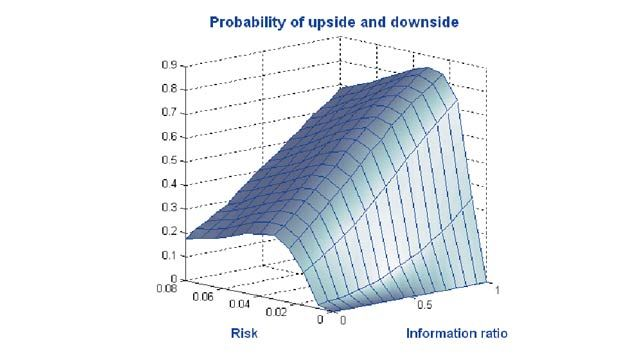Témoignages d'utilisateurs

CAMRADATA Models Dependencies for Quantitative Risk Assessment

Challenge

Rapidly develop quantitative tools for factor analysis, risk analysis, and defensive asset allocation

Solution

Use MATLAB to model complex nonlinear dependencies between assets, liabilities, and economic variables using copulas

Results

• Development time reduced by 90%
• Risk calculated in hours, not weeks
• Diverse skill sets leveraged

“In financial analysis, everything is changing very quickly, and it would be impossible to keep up using any other software tool. With MATLAB we are developing a new solution almost every week. I’ve tried other tools, and I firmly believe that MATLAB is the quickest way of getting to the answers.”Risk-assessment model developed in MATLAB.

For investors, a well-diversified portfolio mitigates risk. To diversify effectively, the investment manager must understand the strength of the relationships between assets in a portfolio. For years, analysts have relied on correlation analysis to quantify this relationship. As a linear measure, however, correlation does not capture the nonlinear relationships that are common in real-world financial markets.

Researchers at CAMRADATA address this problem by using MathWorks tools to build econometric models. Factor analysis helps CAMRADATA understand which economic variables affect asset returns, while copulas help them model factor relationships, particularly during severe market downturns.

“The quantitative tools that we develop with MATLAB enable defensive financial engineering,” says Martyn Dorey, head of quantitative research at CAMRADATA. “With MATLAB we can use copulas, nonlinear math, and complex linkages between assets to really explore what happens when a market crashes.”

Challenge

The CAMRADATA research group, led by Dorey, develops quantitative research for the asset management group, investment consulting clients, and the wider investment industry.

CAMRADATA researchers wanted to calculate the risks between assets and liabilities that portfolios are exposed to during extreme market downturns in order to construct better defensive mechanisms. They needed computational tools that would enable them to identify risks that were common across assets and financial time series. Known as latent factor analysis, this approach requires researchers to look past simple linear relationships between assets and economic variables.

“The demand for customized, numerical, quantitative solutions is increasing exponentially,” notes Dorey. To keep pace with this demand, the researchers sought a development platform that would enable them to develop models quickly and apply them to new problems on very short notice.

Solution

CAMRADATA standardized on MATLAB® and companion toolboxes to develop applications that use copulas to model complex dependencies. Using the t-copula in MATLAB as a starting point, CAMRADATA developed more advanced models that incorpo­rate conditional and asymmetric copulas.

They relied on MATLAB for matrix and vector computations throughout the project. “The MATLAB programming language lends itself very nicely to the language of risk,” says Dorey. “The way you write the math out on a piece of paper as vector matrix calculations translates identically to a line of MATLAB code.”

Using Statistics and Machine Learning Toolbox™, the researchers developed a factor analysis model that shows financial analysts how asset classes are linked through exposure to common factors, such as inflation or the price of oil.

Once they had added asymmetry and tail dependency to the basic correlation parameters, the team used Symbolic Math Toolbox™ to solve maximum likelihood problems and calibrate their models to existing data.

CAMRADATA researchers used MATLAB, Financial Toolbox™, and Optimization Toolbox™ to link and optimize behavioral finance and higher-moments risk budgeting models.

Global Optimization Toolbox and Financial Toolbox were used to develop defensive portfolio designs incorporating option payoff profiles on assets and liabilities. These structured solutions examine the value of using puts and calls to map assets onto liabilities.

For each model, CAMRADATA used MATLAB to build an interface that enables analysts to visualize results and interact with the model using an embedded Microsoft® Excel® spreadsheet.

Using Datafeed Toolbox™, the team gathers time series data from financial data providers such as Bloomberg and has the capability to link to Thomson Reuters Datastream.

CAMRADATA researchers have also used MathWorks tools to develop a universal copula, which enables them to model any seemingly intractable multidimensional relationship. The universal copula has a wide variety of applications, from 3D animation to machine cognition.

Results

• Development time reduced by 90%. “We build all our copulas and models in MATLAB because that is the fastest way for us to deliver a model,” says Dorey. “Using MATLAB we can build a model in one morning. It would take two weeks to write the equivalent code in Visual Basic®.”

• Risk calculated in hours, not weeks. “Calculating a portfolio’s risk going back several years based on underlying stocks can take a week,” Dorey notes. “By using MATLAB to build a model to process risk, we reduced risk-analysis turnaround to less than an hour. For some portfolios, turnaround from a spreadsheet of stock holdings to an automated PowerPoint® presentation takes a minute.”

• Diverse skill sets leveraged. “For complex, nonlinear problems, we need people with a wide range of skills who do not necessarily have a MATLAB background, so help files and technical assistance are key,” Dorey explains. “We recently brought on a plant biologist who researched links between weather and plant disease. Using MATLAB, she is applying that experience to develop new ideas in tail dependency in financial markets.”